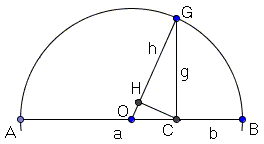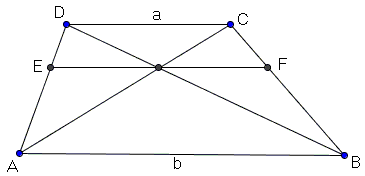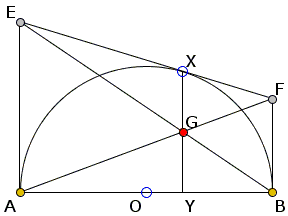# Harmonic Mean in Geometry

The harmonic mean $H(a, b)\;$ of two positive numbers $a\;$ and $b\;$ is defined as

$\displaystyle H(a,b)=\frac{2}{\displaystyle\frac{1}{a}+\frac{1}{b}},$

and equivalently

$\displaystyle\frac{2}{H(a,b)}=\frac{1}{a}+\frac{1}{b},$

or else

$\displaystyle H(a,b)=\frac{2ab}{a+b}.$

It makes a most dramatic appearance in the problem of average speed, but also pops up in various geometric circumstances. Below I collect a few examples.

1. Let $AGB\;$ be a semicircle and $AC = a,\;$ $BC = b.$If $CG \perp AB\;$ and $CH \perp OG\;$ then $GH = H(a, b).$

2. In a trapezoid $ABCD,\;$ let $EF\;$ be the line parallel to the bases $AB\;$ and $CD\;$ through the point of intersection of the diagonals $AC\;$ and $BD:$If $CD = a\;$ and $AB = b,\;$ then $EF = H(a, b).$

It is worth observing that the point of intersection of the diagonals divides $EF\;$ into two equal parts, which leads to another common example:

3. In a 1877 sangaku from the Hyogo prefecture, $AE\;$ and $BD\;$ are both perpendicular to $AB.\;$ $P\;$ is the intersection of $AD\;$ and $BE,\;$ and $CP \perp AB.$If $AE = a\;$ and $BD = b,\;$ then $CP = \displaystyle \frac{1}{2}\cdot H(a, b).$

4. The same motif reemerges in the See-Saw Lemma where $AE,\;$ $BF,\;$ and $EF\;$ are tangent (the latter at $X)\;$ to the semicircle $AXB.$If $AE = a\;$ and $BF = b,\;$ then $XY = H(a, b).$

5. Vladimir Nikolin from Serbia has observed appearance of the harmonic mean in his Rhombus Lemma.Let $AD\;$ be the bisector of $\angle A\;$ in $\Delta ABC,\;$ $B'D||AC\;$ and $C'D||AB.\;$ Then $AB'DC'\;$ is a rhombus and its side $p\;$ is half of the harmonic mean of sides $b = AC\;$ and $b = AB.$

Choosing the angle at $A\;$ to be right leads to a special case where the rhombus becomes a square.

6. Let a square be inscribed into a right triangle as in the diagramThen, if $a\;$ and $b\;$ are the legs of the triangle and p the side of the square, $\displaystyle\frac{1}{p} = \frac{1}{a} + \frac{1}{b}.\;$ In other words, $p = \frac{1}{2}H(a, b).\;$

7. As a consequence of the Rhombus Lemma there is a simple way of constructing the harmonic ratio:In $\Delta ABC,\;$ with $AB = c\;$ and $AC = b,\;$ draw $AD\;$ - the bisector of $\angle A.\;$ At $D\;$ erect a perpendicular to $AD\;$ and let $E\;$ be its intersection with either $AB\;$ or $AC.\;$ Then $AE = H(b, c).$• The Means
• Averages, Arithmetic and Harmonic Means
• Expectation
• The Size of a Class: Two Viewpoints
• Averages of divisors of a given integer
• Family Statistics: an Interactive Gadget
• Averages in a sequence
• Arithmetic and Geometric Means
• Geometric Meaning of the Geometric Mean
• A Mathematical Rabbit out of an Algebraic Hat
• AM-GM Inequality
• The Mean Property of the Mean
•